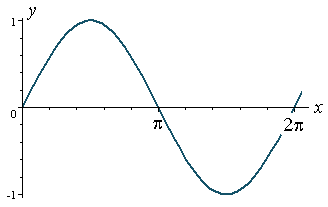Search IntMath
Close

# Friday Math Movie - Sine Wave to Square Wave using Fourier Series

By Murray Bourne, 14 Nov 2008

We learned about sine waves in elementary trigonometry:The idea behind the Fourier Series is to add sine curves with different amplitudes and frequencies and the resulting curve can be either a square wave, a sawtooth wave or many other interesting periodic shapes. You can see more on this concept in this Introduction to Fourier Series.

This week's movie begins with a pure sine wave tone and then the other sine curves are added to it to produce something very close to a square wave (with the odd wiggle here and there) as the number of added curves becomes very large.

Warning: The final square wave tone may be pretty loud.

### Comment Preview

HTML: You can use simple tags like <b>, <a href="...">, etc.

To enter math, you can can either:

1. Use simple calculator-like input in the following format (surround your math in backticks, or qq on tablet or phone):
a^2 = sqrt(b^2 + c^2)
(See more on ASCIIMath syntax); or
2. Use simple LaTeX in the following format. Surround your math with $$ and $$.
$$\int g dx = \sqrt{\frac{a}{b}}$$
(This is standard simple LaTeX.)

NOTE: You can mix both types of math entry in your comment.